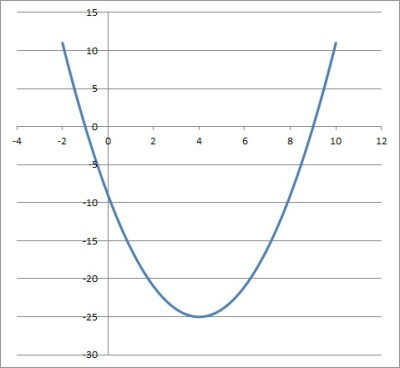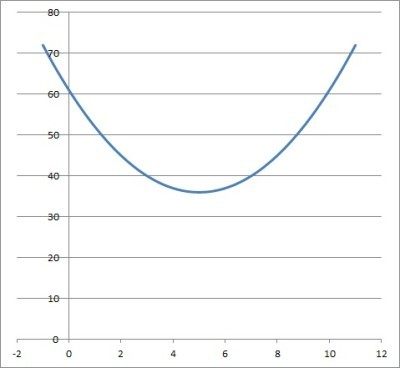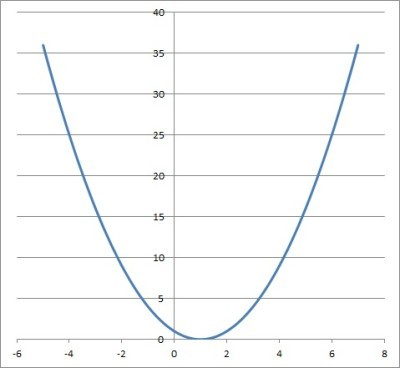# How To Complete The Square To Solve A Quadratic Equation

Completing the square is a method that can help us to solve a quadratic equation.  It also has other uses in mathematics, so it is worth knowing how to do it.

So, how do you complete the square to solve a quadratic equation?  To complete the square, first rearrange the quadratic equation so it has the form x2 + Bx = C. Next, add B2 / 4 to both sides. Then, factor the left side as (x + B/2)2. Now, take the square root of both sides. Finally, subtract B/2 from both sides to get the solutions of the quadratic equation.

Of course, completing the square is used to derive the quadratic formula (the formula is a shortcut for this method!)

In this article, we’ll talk about how to complete the square and how to use this method to solve a quadratic equation.  Then we’ll circle back to talk about another use of completing the square.

Let’s get started.

## How To Complete The Square

To complete the square for a quadratic equation in the form ax2 + bx + c = 0, take these steps:

• First, rearrange the equation so that the quadratic and linear terms are on the left side, and the constant term is on the right side.  So, convert to the form x2 + Bx = C (you may need to divide by a on both sides of the equation to get a coefficient of 1 for the x2 term).
• Next, calculate the value of B / 2 (that is, take half of B).
• Then, square the result from the last step, giving you (B / 2)2 = B2 / 4.
• Now, add the result from the last step to both sides of the equation (that is, add B2 / 4 to both sides of the equation).  At this point, the square on the left side is complete, but not factored.
• Next, combine like terms (constants) on the right side of the equation to get C + B2 / 4.
• Then, factor the left side as a perfect square trinomial: (x + B/2)2.
• Now, take the square root of both sides of the equation.  This leaves us with x + B/2 on the left side.
• Finally, subtract B / 2 from both sides of the equation.  This leaves us with x on the left side.

(Note that B and C may not be the same as b and c in the original equation).

Note that completing the square will give us two distinct solutions (either two real or two complex solutions) unless we have a double real root.

Let’s take a look at some examples to see how this works.

### Example 1: Completing The Square

• x2 – 8x – 9 = 0

We will complete the square to solve.

First, we rearrange the equation so that it has the form x2 + Bx = C.  Here, this means we add 9 to both sides to get:

• x2 – 8x = 9

Remember that if we have x2 + Bx = C, then we take half of B, square it, and then add that amount to both sides of the equation (that is, add B2 / 4 to both sides).

Here, we have B = -8, so we add B2 / 4 = (-8)2 / 4 = 64 / 4 = 16 to both sides of the equation:

• x2 – 8x + 16 = 9 + 16
• x2 – 8x + 16 = 25

Now we have completed the square on the left side of the equation.  Because of this, we have a perfect square trinomial of the form

• x2 – 2Ax + A2

on the left side, with A = 4.

This perfect square trinomial factors as:

• x2 – 2Ax + A2 = (x – A)(x – A)

or

• x2 – 2Ax + A2 = (x – A)2

Applying this formula with A = 4 gives us:

• x2 – 8x + 16 = 25
• (x – 4)2 = 25

Now we can take the square root of both sides to get:

• √(x – 4)2 = √25
• x – 4 = √25

Since +5 and -5 are both square roots of 25, we get two different solutions: x = 4 + 5 and x = 4 – 5.  (Note: this means the discriminant in the original quadratic equation was positive).

So the two solutions are x = -1 and x = 9.  You can see this in the graph of the quadratic below.The graph of the quadratic y = x2 -8x – 9. It has zeros at x = -1 and x = 9, which are the solutions to the corresponding quadratic equation.

### Example 2: Completing The Square

• x2 – 10x + 61 = 0

We will complete the square to solve.

First, we rearrange the equation so that it has the form x2 + Bx = C.  Here, this means we subtract 61 from sides to get:

• x2 – 10x = -61

Remember that if we have x2 + Bx = C, then we take half of B, square it, and then add that amount to both sides of the equation (that is, add B2 / 4 to both sides).

Here, we have B = -10, so we add B2 / 4 = (-10)2 / 4 = 100 / 4 = 25 to both sides of the equation:

• x2 – 10x + 25 = -61 + 25
• x2 – 10x + 25 = -36

Now we have completed the square on the left side of the equation.  Because of this, we have a perfect square trinomial of the form

• x2 – 2Ax + A2

on the left side, with A = 5.

This perfect square trinomial factors as:

• x2 – 2Ax + A2 = (x – A)(x – A)

or

• x2 – 2Ax + A2 = (x – A)2

Applying this formula with A = 5 gives us:

• x2 – 10x + 25 = -36
• (x – 5)2 = -36

Now we can take the square root of both sides to get:

• √(x – 5)2 = √-36
• x – 5 = √-36

Since +6i and -6i are both square roots of -36, we get two different solutions: x = 5 + 6i and x = 5 – 6i.

So the two solutions are x = 5 + 6i and x = 5 – 6i, which are complex conjugates (meaning the real parts are the same, but the imaginary parts are different).  (Note: this means the discriminant in the original quadratic equation was negative).

You can see the graph of the quadratic below (the solutions do not appear on the graph, since they are complex).The graph of the quadratic y = x2 -10x + 61 It has zeros at x = 5 + 6i and x = 5 – 6i, which are solutions to the corresponding quadratic equation (they do not appear on the graph because they are complex numbers).

### Example 3: Completing The Square

• x2 – 2x + 1  = 0

We will complete the square to solve.  However, this is sort of trick question, since the square is already complete on the left side!

Look: on the left side, we already have a perfect square trinomial of the form

• x2 – 2Ax + A2

on the left side, with A = 1.

This perfect square trinomial factors as:

• x2 – 2Ax + A2 = (x – A)(x – A)

or

• x2 – 2Ax + A2 = (x – A)2

Applying this formula with A = 1 gives us:

• x2 – 2x + 1 = 0
• (x – 1)2 = 0

Now we can take the square root of both sides to get:

• √(x – 1)2 = √0
• x – 1 = 0
• x = 1

Since 0 only has one square root (which is zero), there is only one solution to this quadratic equation: the double real root x = 1.  (Note: this means the discriminant in the original quadratic equation was zero).

You can see this in the graph below (the vertex of the parabola rests on the x-axis, and the x-coordinate is the double real root of the quadratic equation).The graph of the quadratic y = x2 -2x + 1 It has a real zero at x = 1 (a double real root), which is the solution to the corresponding quadratic equation (in this case, the parabola just touches the x-axis, with its vertex resting on the line y = 0),

## What Do You Use Completing The Square For?

Besides solving a quadratic equation, we can also use completing the square to convert a circle equation to the standard form

• (x – a)2 + (y – b)2 = r2Completing the square to convert a circle equation to standard form makes it easy for us to find the center and radius. This makes it much easier to graph the circle.

This standard circle equation makes it easier to graph, since we can read the center and radius directly from the resulting circle equation:

• Center = (a, b)

Let’s try some examples to see how this method works.

### Example 1: Completing The Square To Convert A Circle To Standard Form (y Variable Only)

Consider the following circle equation:

• x2 – 2x + 1 + y2 + 4y = 5

We can see that the square is already complete for the x-variable, since we can factor x2 – 2x + 1 as (x – 1)2:

• (x – 1)2 + y2 + 4y = 5

Now we need to complete the square for the y variable.  On the left side, we have y2 + By = C with B = 4.

Remember that if we have y2 + Bx = C, then we take half of B, square it, and then add that amount to both sides of the equation (that is, add B2 / 4 to both sides).

Here, we have B = 4, so we add B2 / 4 = (4)2 / 4 = 16 / 4 = 4 to both sides of the equation:

• (x – 1)2 + y2 + 4y + 4 = 5 + 4
• (x – 1)2 + y2 + 4y + 4 = 9
• (x – 1)2 + (y + 2)2 = 32

For the last step, we factored y2 + 4y + 4 as (y + 2)2.  This gives us the equation of the circle in standard form.

So, we have a circle with center (a, b) = (1, -2) and radius r = 3.

### Example 2: Completing The Square To Convert A Circle To Standard Form (x Variable Only)

Consider the following circle equation:

• x2 – 12x + y2 – 6y + 9 = 28

We can see that the square is already complete for the y-variable, since we can factor y2 – 6y + 9 as (y – 3)2:

• x2 – 12x + (y – 3)2 = 28

Now we need to complete the square for the x variable.  On the left side, we have x2 + Bx = C with B = -12.

Remember that if we have y2 + Bx = C, then we take half of B, square it, and then add that amount to both sides of the equation (that is, add B2 / 4 to both sides).

Here, we have B = -12, so we add B2 / 4 = (-12)2 / 4 = 144 / 4 = 36 to both sides of the equation:

• x2 – 12x + 36 + (y – 3)2 = 28 + 36
• x2 – 12x + 36 + (y – 3)2 = 64
• (x – 6)2 + (y – 3)2 = 82

For the last step, we factored x2 – 12x + 36 as (x – 6)2.  This gives us the equation of the circle in standard form.

So, we have a circle with center (a, b) = (6, 3) and radius r = 8.

### Example 3: Completing The Square To Convert A Circle To Standard Form (Both x & y Variables)

Consider the following circle equation:

• x2 + 24x + y2 + 10y = 0

We will need to add (24/2)2 = 122 = 144 to both sides to complete the square for the x-variable:

• x2 + 24x + 144 + y2 + 10y = 0 + 144
• (x + 12)2 + y2 + 10y = 144

We will also need to add (10/2)2 = 52 = 25 to both sides to complete the square for the y-variable:

• (x + 12)2 + y2 + 10y + 25 = 144 + 25
• (x + 12)2 + (y + 5)2 = 169
• (x + 12)2 + (y + 5)2 = 132

This gives us the equation of the circle in standard form.

So, we have a circle with center (a, b) = (-12, -5) and radius r = 13.

## Conclusion

Now you know how to complete the square to solve a quadratic equation.  You also know how to use the method of completing the square to convert a circle equation into standard form.